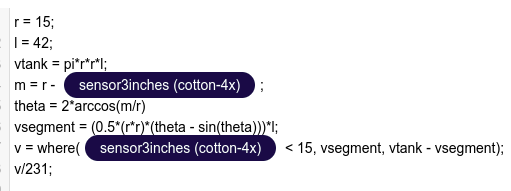# How To: Calculate a Complex Value (gallons in a horizontal cylinder)?

Team,

I don’t want this calculation to be a synthetic variable - or at least I don’t think so.

So, where would I do a calculation like this - as an Ubifunction - that maybe seems like overkill?

Or is there another place I can put some small math (coSine would be needed)?

Thanks!

Hello, @marlanw

Thanks for reaching out,

We had a similar requirement in the past and it was done using a synthetic variable, based on the formula available here, the readings of the tank level sensor indicated the level of liquid but the volume was calculated using the formula. Keep in mind that the synthetic variables editor allows mathematical expressions as indicated here.

When using the Ubifuncton, you may need to make an API request on a regular basis to obtain the last value make the calculations, and then send the value to a new variable.

Regards,

-Santiago

Thanks Santiago - the formula is behind an intercom account and I don’t have intercom. I have seen several formulas for doing this, but would love to see what this one says. I’ll go ahead and use the synthetic variable then. I worry about using them too much.

Hello, @marlanw

I’m sorry I pasted the wrong link but I’ve just fixed it on the original response
The website nonetheless is this one.

On synthetics - I ASSUME that the LAST value on the stack is what is returned. I could not find a basic how-to or syntax for the synthetic variables - OR the language they use. Makes debug very hard.

Here is my synthetic variable calc - which is NOT validating:
length = 15000
height = 10000
specialheight = where( liquidheight > radius , height - liquidheight , radius - liquidheight )
specialvolume = rsquare * ( thetaradians - sin( thetaradians )) * length / 2
volumemm3 = where( liquidheight > radius , ( rsquare * pi * length) - specialvolume , specialvolume )
volumegallons = volumemm3 * 0.00000026417
specialheight ## put here as a spoof so I could save the formula in the synth variable

Just an idea - it looks like your expression validator isn’t tracking that the result of the {{sensorreading}} is going through the arcos() function. If you put the value thetaradians at the end of the list of calculations, the validator will not validate. So specialheight is the last variable to validate.

Hello team - I noticed the Help Center doc uses “arcos” and the PDF uses “arccos”. Funny thing - when I used arccos the darn thing passes validation.

I might suggest fixing the Help Center doc.

Hello, @marlanw

This is an example of a similar calculation based on the formula found on the website. there the arccos() function is used just like suggested on the formula, for a more updated reference on synthetic variables please check this link. The value returned is indeed the last line.We are glad that it works now and we can definitively update or documentation and perhaps post an example of a calculation for a tank’s volume.

Regards,

-Santiago

1 Like